### Introduction

This case is inspired by the experimental work of T. H. Kuehn and R. J. Goldstein  who investigated the buoyancy driven flow of nitrogen in a horizontal cylindrical cavity. A two dimensional cross section of one of their experiments is shown in Figure 1.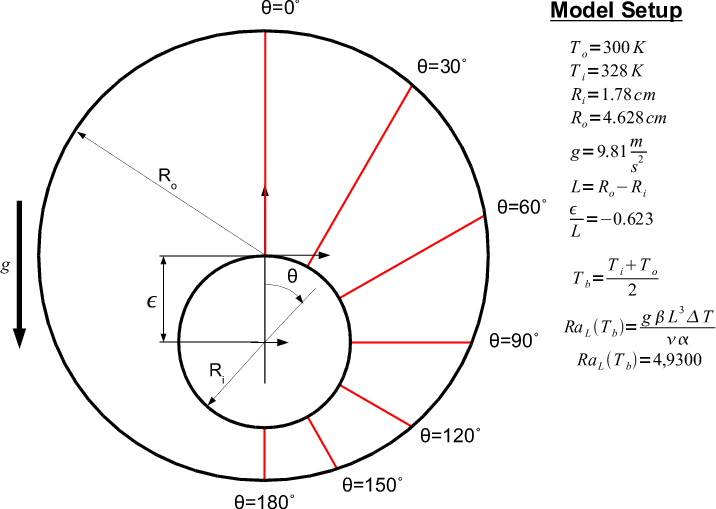Figure 1

This geometry consists of two cylinders, a large and small, one inside the other. The smaller cylinder acts as a heat source and is placed inside the larger cylinder to promote fluid motion. Kuehn and Goldstein investigated several different cavity arrangements including different cavity pressures and positions of the inner cylinder relative the the outer cylinder.

### Model Setup

The experimental setup of Kuehn and Goldstein's paper has been selected for modeling. The geometry for this case is shown in Figure 1. Kuehn and Goldstein present detailed temperature data along each red line of Figure 1. According to their comments, the flow is laminar with a Rayleigh number of 49,300 and a Prandtl number of 0.706. The flow field inside the cavity is assumed to be two dimensional for this modeling effort. The model domain was discretized using polygonal mesh topology consisting of 5,849 control volumes and 34,140 control volume faces (see Figure 2).Figure 2

The fluid properties of nitrogen were used in the model. Nitrogen was assumed to be an ideal gas. The cavity pressure was assumed to be constant at atmospheric pressure. The density was assumed to vary according to the ideal gas law. The laminar viscosity was assumed to follow Sutherland's law. A 4th order polynomial for the specific heat of nitrogen was used. Standard gravity of 9.81 m/s2 was assumed to act in the direction indicated in Figure 1. The incompressible SIMPLE method was used to couple the momentum and continuity equations. A second order up-winding method was used for the velocity and temperature fields. The inner cylinder was given a constant temperature Tinner=328 K and the outer cylinder was fixed at Touter=300 K.

### Model Results

Figure 3 shows the general flow structure predicted. The color contours are of temperature Kelvin and the arrows indicate the direction of the fluid motion. In this image you can see that the nitrogen is being heated by the inner cylinder and begins to rise up. Note that the geometry and the flow field are symmetric about the vertical centerline of the cavity. Kuehn and Goldstein provided detailed nitrogen temperatures along radial lines extending from the center of the inner cylinder to the surface of the outer cylinder, see the red lines in Figure 1. For presentation purposes the length of the radial lines was normalized based on the total distance. Figures 4-11 below show the model fluid temperature predictions compared to the experimental data collected.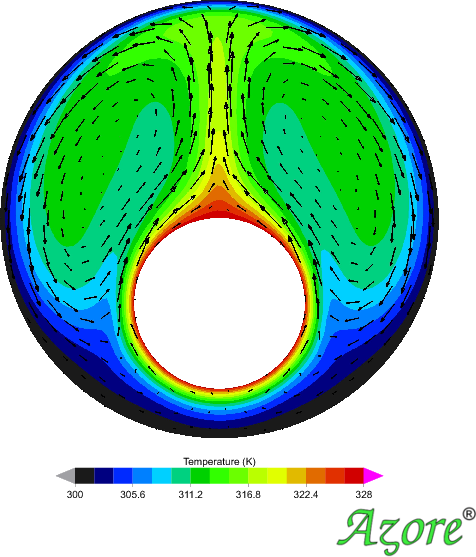Figure 3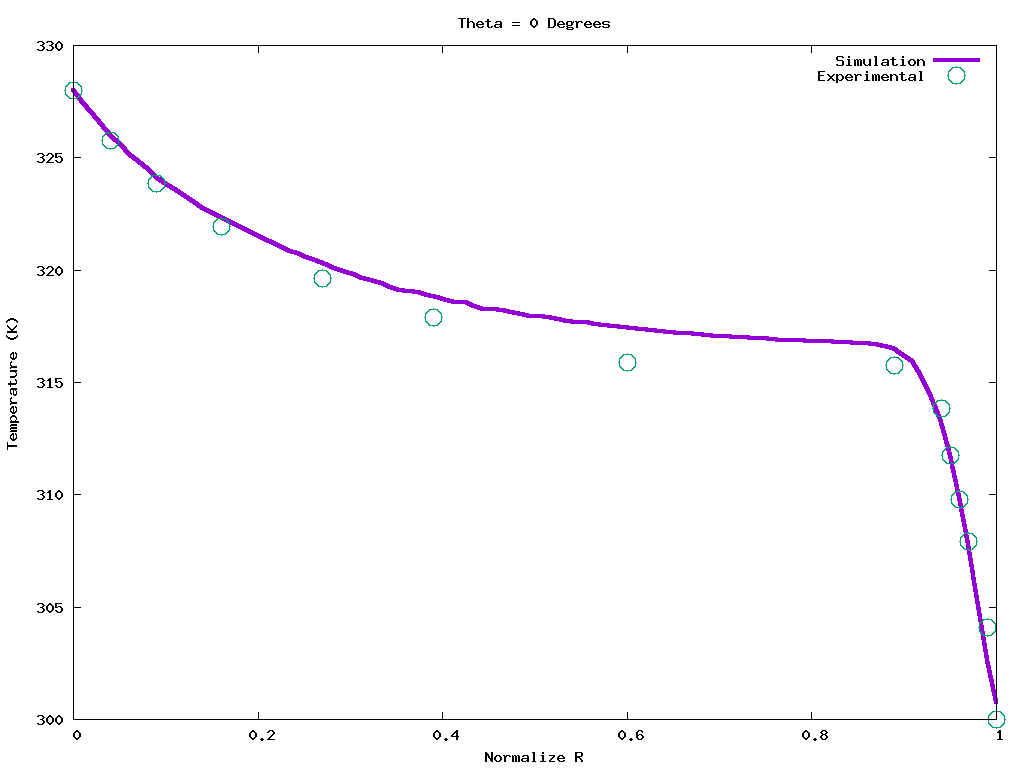Figure 4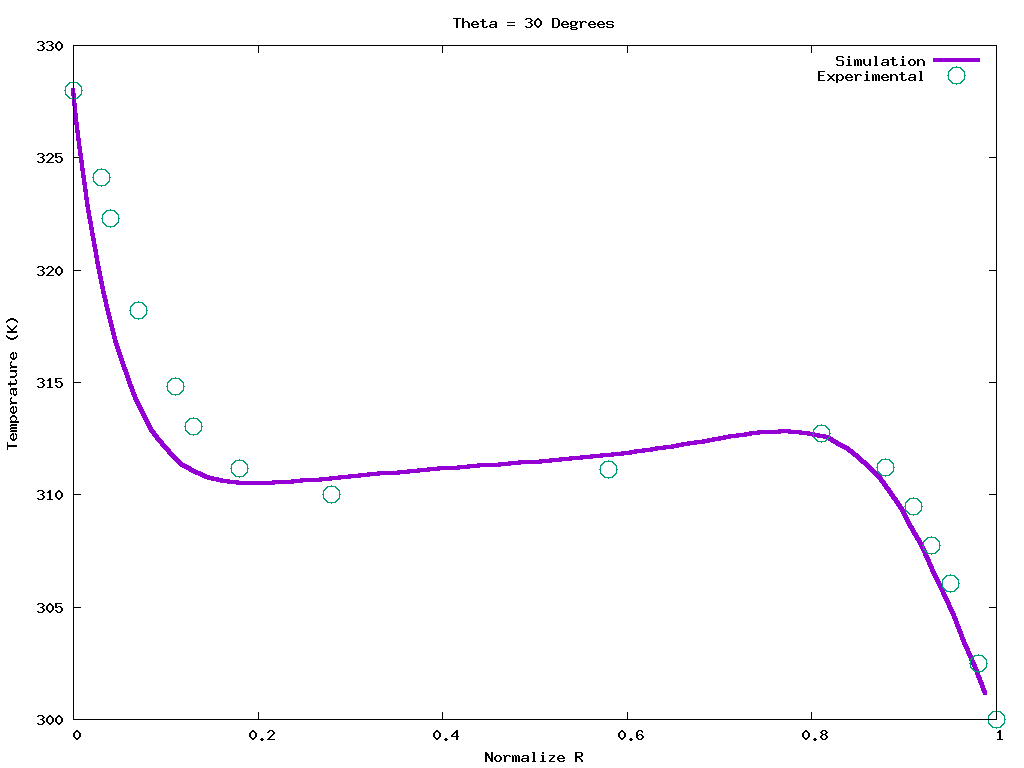Figure 5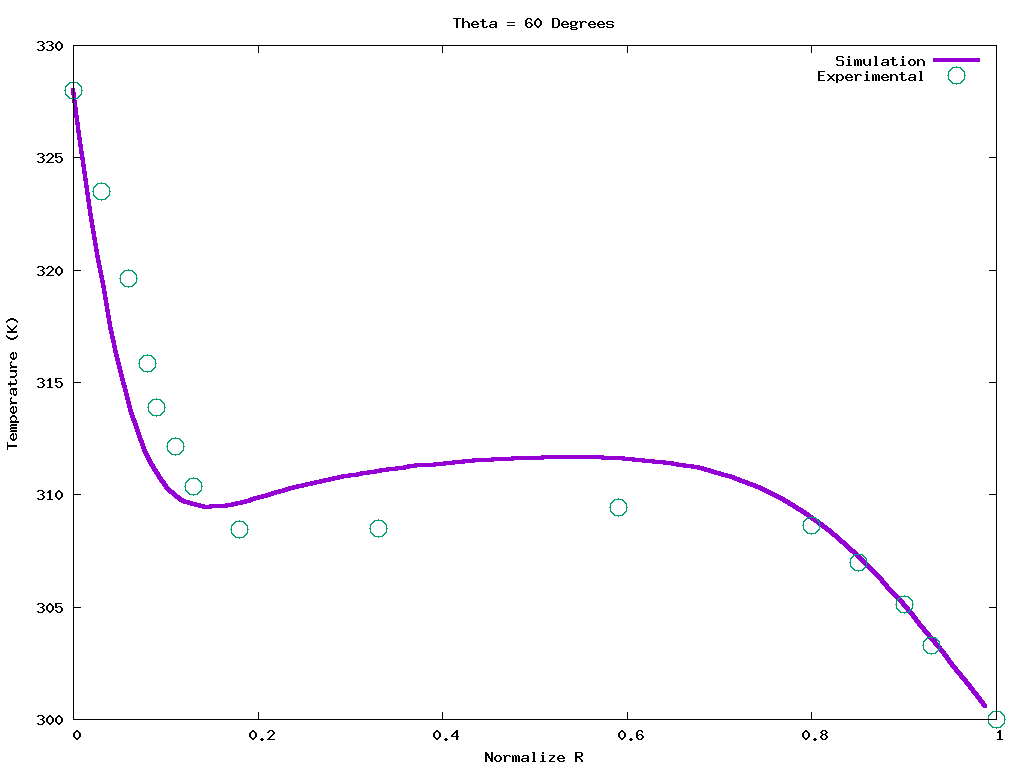Figure 6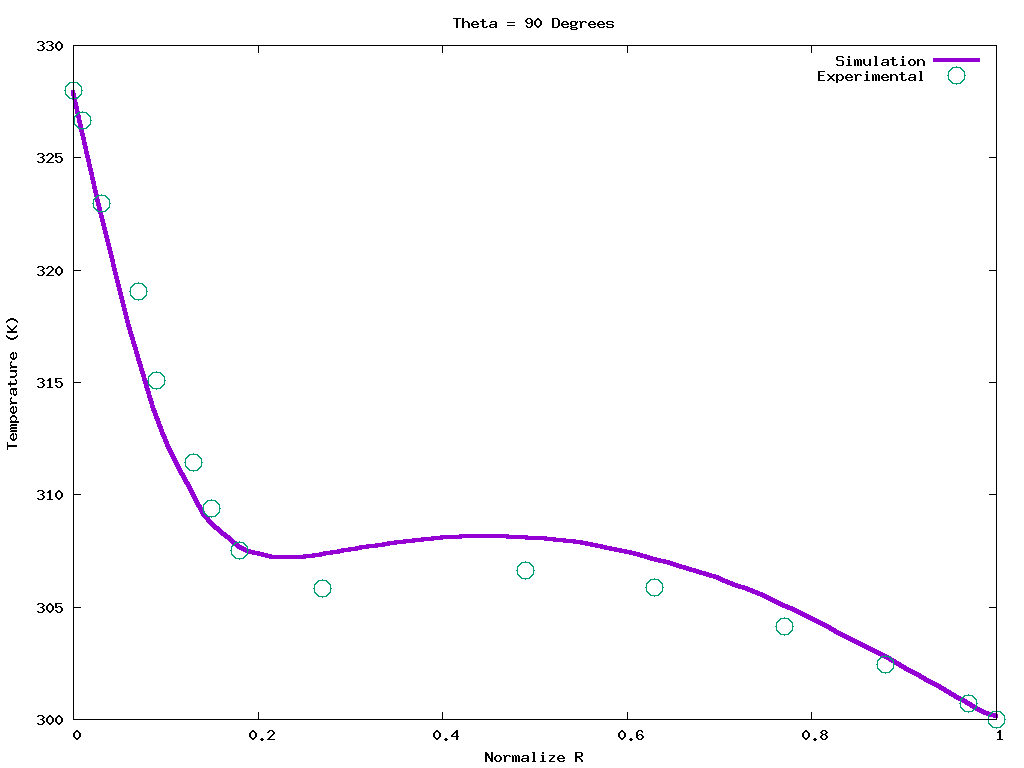Figure 7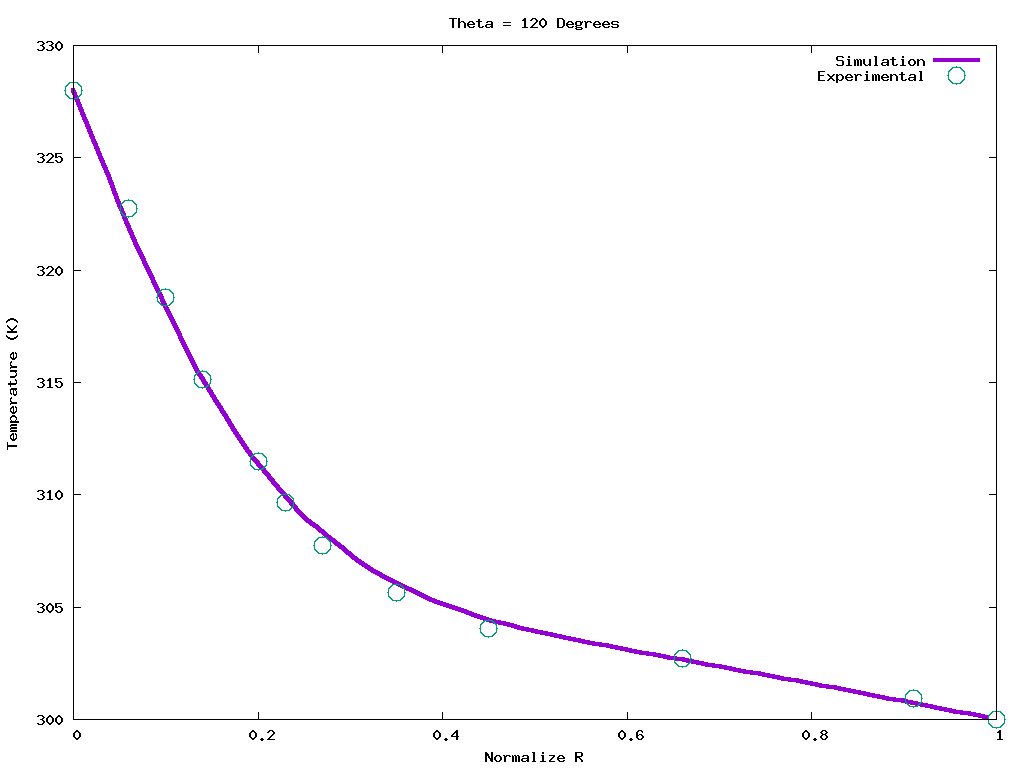Figure 8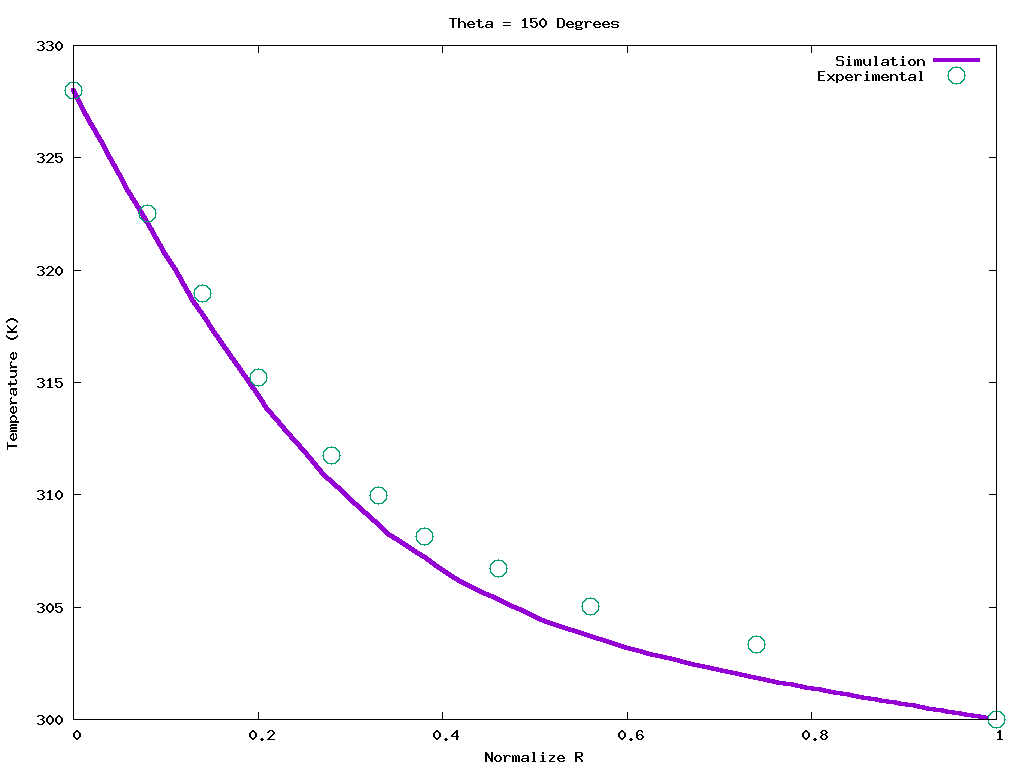Figure 9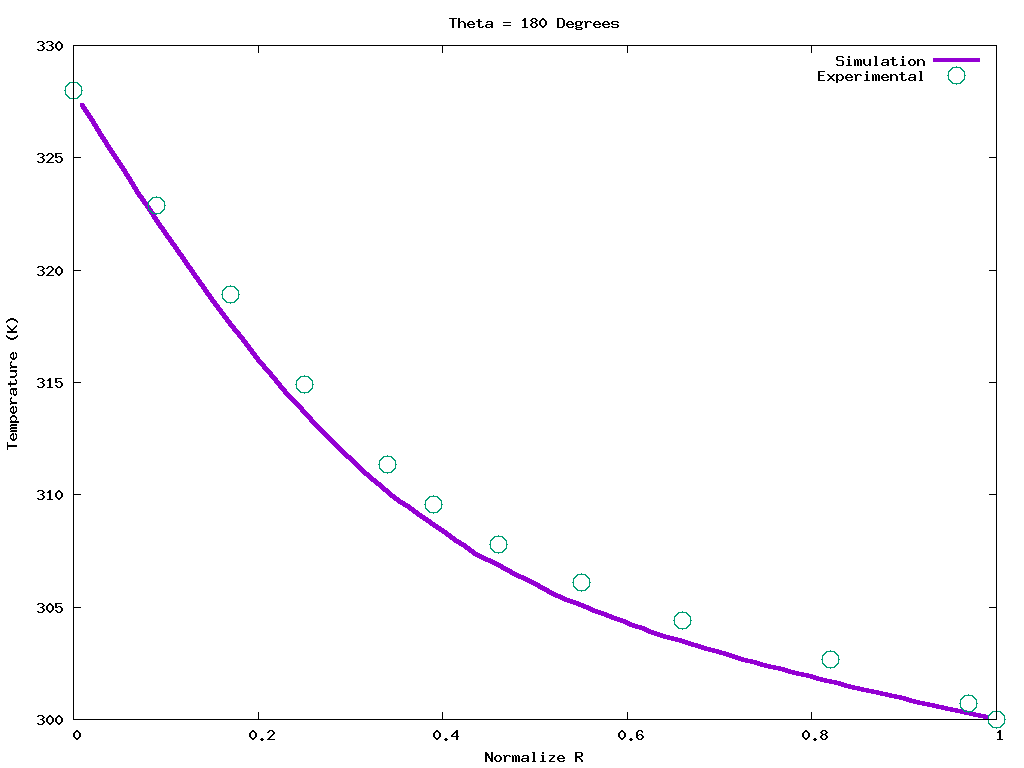Figure 10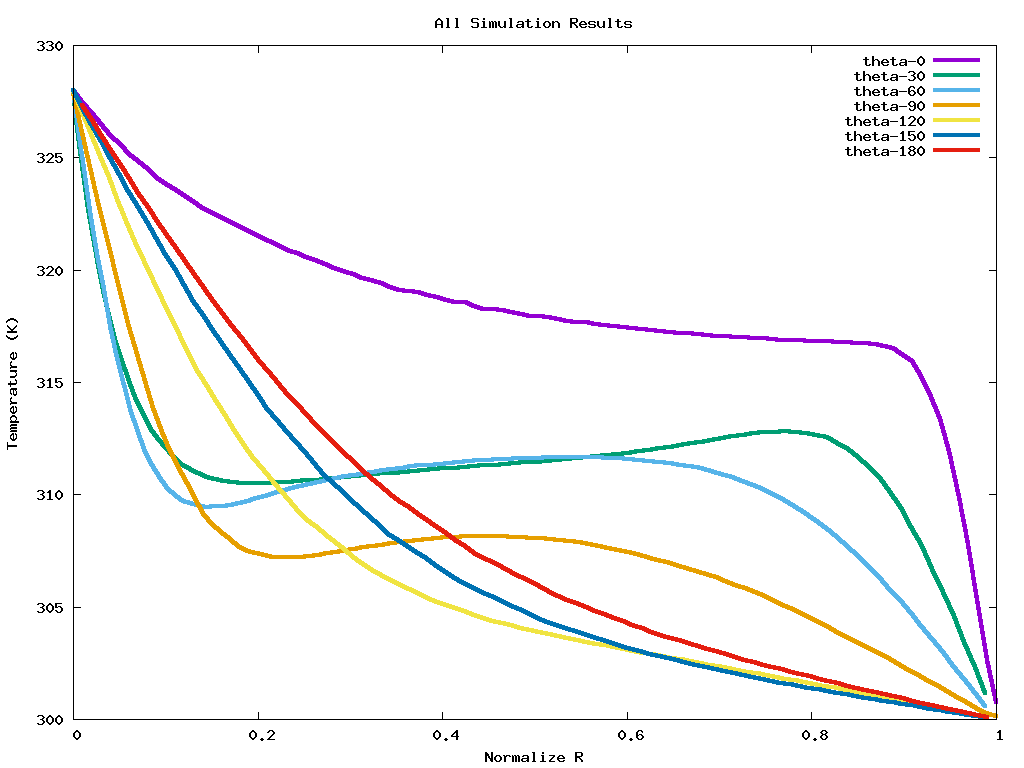Figure 11

  T. H. Kuehn and R. J. Goldstein, An experimental study of natural convection heat transfer in concentric and eccentric horizontal cylindrical annuli, Journal of Heat Transfer, Vol. 100, (1978 November), 635–640.